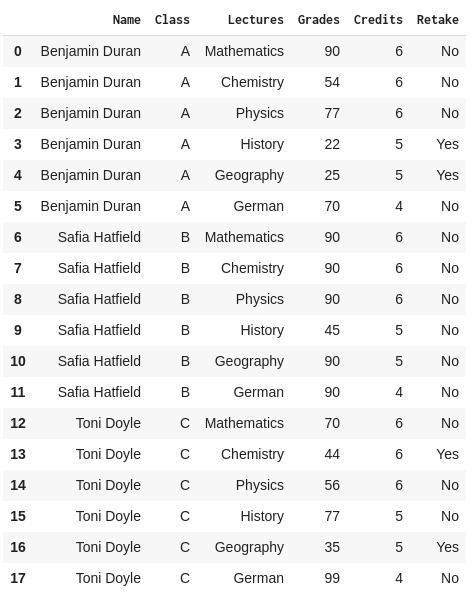# How to Access an Element in PandasBefore we start: This Python tutorial is a part of our series of Python Package tutorials. The steps explained ahead are related to the sample project introduced here.
Learn how to access an element in a Pandas Dataframe using the iat and at functions. Using the Pandas library in Python, you can access elements, a single row or column, or access multiple elements, rows and columns and visualize them. Let’s see how.

First, we need to read in our CSV file that we will be working with:

`Report_Card = pd.read_csv("Report_Card.csv")`If we wanted to access an element, say a certain grade of a student, we could use either the iat or at function. For example, we can use the following function to get the Mathematics grade for Benjamin using the at function:

`Report_Card.loc.at["Grades"]`

This line of code will return us the value 90. Let’s break it down to see what each function does to see how the value 90 is returned. The first function Report_Card.loc returns us the following Pandas.Series object:Then we apply at[“Grades”] to this Panda.Series object, which returns us the value corresponding to the Grades column. Similar to the loc and iloc functions for accessing row and column data, here we make use of Pandas’ at function to access an element.

Pandas also provides an iat function, which we can use to index the value we want using the integer-valued indices. The following code using the iat function will also return us the value of 90:

`Report_Card.loc.iat`

Since column indices start from 0, here the value 3 corresponds to the index of the column named Grade.

There is also a shorthand notation that we can use with iat and at functions. Instead of using the loc function to get the row as a Series object, we can directly use the following code snippets:

```Report_Card.at[0,"Grades"]
Report_Card.iat[0,3]```

Sometimes we may want to turn our Dataframe, or a part of it, into a 2D array for use with different use cases. Pandas offer us a function called to_numpy() which turns the DataFrame that it is used on into a 2D numpy array:

`matrix_res = Report_Card.to_numpy()`

The above code snippet will return us a 2D array of the same structure as our DataFrame, but with column names removed. Now we can just index it the same way we index regular Python 2D lists, and simply use matrix_res to get the value 90.

#### Next steps

Now that you know how to access an element in DataFrames using Python’s Pandas library, let’s move on to other things you can do with Pandas:

#### Get Pre-compiled Python Packages For Data Science, Web Development, Machine Learning, Code Quality And Security

If you’re one of the many engineers using Python to build your algorithms, ActivePython is the right choice for your projects Get The Machine Learning Packages You Need – No Configuration Required. We’ve built the hard-to-build packages so you don’t have to waste time on configuration…get started right away! Learn more about ActivePython here.

With deep roots in open source, and as a founding member of the Python Foundation, ActiveState actively contributes to the Python community. We offer the convenience, security and support that your enterprise needs while being compatible with the open source distribution of Python.